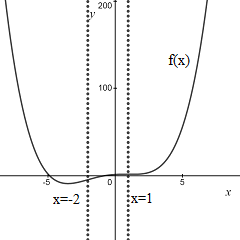# Sketch the graph for a single function that has all of the properties listed. f''(x) greater than...

## Question:

Sketch the graph for a single function that has all of the properties listed.

{eq}f''(x) > 0 {/eq} on {eq}(-\infty, -2) {/eq} and {eq}(1, \infty) {/eq}

## Concavity of a Function:

A function of one variable {eq}f(x) {/eq} is said "concave up" in the open intervals where its second derivative is positive.

The function is said "concave down" in the open intervals where the second derivative is negative.

From input data, we know that a function has the following properties:

{eq}f''(x) > 0 {/eq} on {eq}(-\infty, -2) {/eq} and {eq}(1, \infty). {/eq}

This means that the function is concave up in the interval {eq}(-\infty, -2) {/eq} and in the interval {eq}(1, \infty). {/eq}

A function with these properties has its second derivative equal to

{eq}f''(x) = ( x+2)(x-1) = x^2+x-2. {/eq}

Then, the unknown function is found by integrating twice its second derivative

{eq}\displaystyle f'(x) = \frac{1}{3} x^3+\frac{1}{2}x^2-2x + C \\ \displaystyle f(x) = \frac{1}{12} x^4+\frac{1}{6}x^3-x^2 + Cx + D. {/eq}

A sketch of the function when {eq}C=D=1 {/eq} is reported in the figure below.